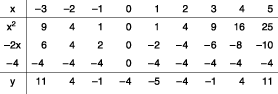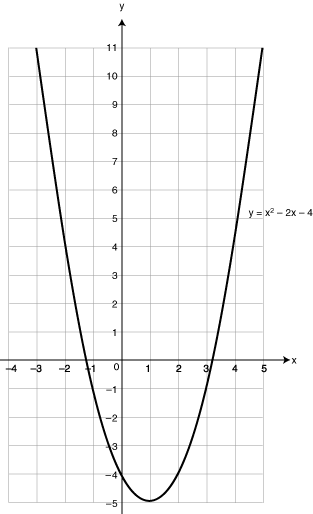Definition: quadratic equation from Philip's Encyclopedia

Algebraic equation in which the highest exponent of the variable is 2; an equation of the second degree. A quadratic equation has the general form ax2 + bx + c = 0, where a, b and c are constants. It has, at most, two solutions (roots), given by the formula x = [-b ± √(b2 - 4ac)]/2a.

from The Hutchinson Unabridged Encyclopedia with Atlas and Weather Guide

In mathematics, a polynomial equation of second degree (that is, an equation containing as its highest power the square of a variable, such as x2). The general formula of such equations is

ax2 + bx + c = 0

in which a, b, and c are real numbers, and only the coefficienta cannot equal 0.

In coordinate geometry, a quadratic function represents a parabola.

Some quadratic equations can be solved by factorization (see factor (algebra)), or the values of x can be found by using the formula for the general solution

x = [−b + √(b2 − 4ac)]/2a or

x = [−b − √(b2 − 4ac)]/2a

Depending on the value of the discriminant, b2 − 4ac, a quadratic equation has two real, two equal, or two complex roots (solutions). When b2 − 4ac > 0, there are two distinct real roots. When b2 − 4ac = 0, there are two equal real roots. When b2 − 4ac < 0, there are two distinct complex roots.

Solving quadratics by factorizing If a quadratic equation can be factorized, it can be solved. For example, to solve x2 + x − 6 = 0, the equation should be factorized to give:

(x + 3)(x − 2) = 0

There are now two brackets multiplied together to give 0. This means that one of them must be 0. So either x + 3 = 0 or x − 2 = 0. Therefore, x = −3 or x = 2.

Drawing the graphs of quadratics Quadratic equations always plot parabolas, whereas linear equations plot straight lines. For example, to draw the graph of y = x2 − 2x − 4, the table should be completed:and then the graph plotted:From the graph, the solution to the equation y = x2 − 2x − 4 is

x = −1.2 or x = 3.2

Completing the square can solve other quadratics.

essays

Multiplying out brackets and factorizing

Out for the Count – Problem Solving Using Quadratic Equations

Algebra: simultaneous equations

Simplification of surds

SOS Mathematics: Algebra© RM, 2018. All rights reserved.

Related Articles

The Macmillan Encyclopedia

An algebraic equation in which the greatest power of the variable is two. It is usually written in the form ax 2 + bx + c = 0, in...